SSC BOARD PAPERS IMPORTANT TOPICS COVERED FOR BOARD EXAM 2024

### Practice Set 7.4 Mensuration Class 10th Mathematics Part 2 MHB Solution

Practice Set 7.4
1. In figure 7.43, A is the centre of the circle. ∠ ABC = 45° and AC = 7√2 cm. Find the…
2. In the figure 7.44, O is the centre of the circle. M (arc PQR) = 60° OP = 10 cm. Find…
3. In the figure 7.45, if A is the centre of the circle. ∠ PAR = 30°, AP = 7.5, find the…
4. In the figure 7.46, if O is the centre of the circle, PQ is a chord. ∠ POQ = 90°, area…
5. A chord PQ of a circle with radius 15 cm subtends an angle of 60° with the centre of…
###### Practice Set 7.4
Question 1.

In figure 7.43, A is the centre of the circle. ∠ ABC = 45° and AC = 7√2 cm. Find the area of segment BXC.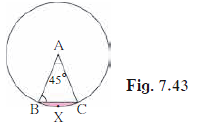From the property, we know that, If two sides of a triangle are equal then their corresponding angles are also equal.

So, as AB = AC,

⇒ ∠ ABC = ∠ ACB = 45°

As the sum of angles of a triangle is equal to 180°

⇒∠ ABC + ∠ ACB + ∠ BAC = 180°

⇒45° + 45° + ∠ BAC = 180°

⇒90° + ∠ BAC = 180°

⇒ ∠ BAC = 90°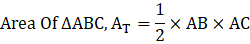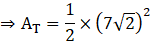⇒ AT = 49 sq.cm

Area of the sector = one-fourth of a circle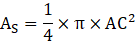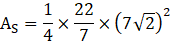⇒ AS = 77 sq.cm

Area of the shaded region, AR = AS - AT

⇒ AR = 77 – 49

⇒ AR = 28 sq.cm

∴ The area of the shaded region is 28 sq. cm.

Question 2.

In the figure 7.44, O is the centre of the circle. M (arc PQR) = 60° OP = 10 cm.

Find the area of the shaded region. (π = 3.14, √3 = 1.73)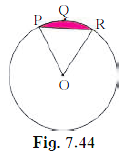Since the angle subtended at centre is 60°

And by the property, if two sides of a triangle are equal then their corresponding angles are also equal.

⇒ ∠ ORP = ∠ OPR

As the sum of all internal angles of a triangle is equal to 180°

⇒ ∠ ORP = ∠ OPR = 60°

⇒ Δ OPR is an equilateral triangle.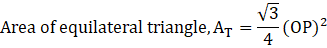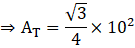⇒ AT = 43.25 sq. cm

Area Of Sector (O-PQR), AS is given as: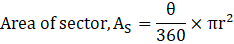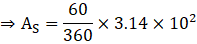⇒ AS = 52.33 sq.cm

Area of shaded region, AR = As – AT

⇒ AR = 52.33 – 43.25

⇒ AR = 9.08 sq.cm

∴ Area of shaded region is 9.08 sq.cm

Question 3.

In the figure 7.45, if A is the centre of the circle. ∠ PAR = 30°, AP = 7.5, find the area of the segment PQR. (π = 3.14)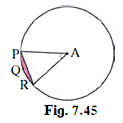Radius of circle, r = 7.5 cm

∠ PAR = θ = 30°

Area(A – PQR), AS: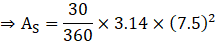⇒ AS = 14.71 sq.cm

Also,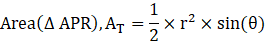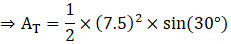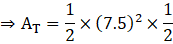⇒ AT = 14.06 sq. cm

Area of segment PQR, AR = AS - AT

⇒ AR = 14.71 – 14.06 = 0.6562 sq.cm

∴ Area of shaded region is 0.6562 sq.cm

Question 4.

In the figure 7.46, if O is the centre of the circle, PQ is a chord. ∠ POQ = 90°, area of shaded region is 114 cm2, find the radius of the circle. (π = 3.14)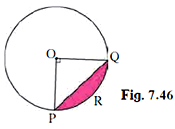Area Of shaded region, AR = 114 sq.cm

Area of sector (O-PRQ),AS = one-fourth of area of circle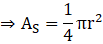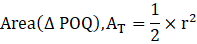Area of Shaded Region, AR = AS – AT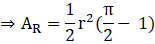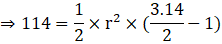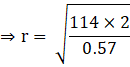⇒ r = 20 cm

∴ Radius of the circle is 20 cm

Question 5.

A chord PQ of a circle with radius 15 cm subtends an angle of 60° with the centre of the circle. Find the area of the minor as well as the major segment. (π = 3.14)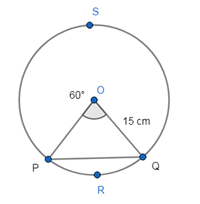Radius of circle, r = 15cm

Central angle, θ = 60°

Since the angle subtended at centre is 60°

And by the property, if two sides of a triangle are equal then their corresponding angles are also equal.

⇒ ∠ OQP = ∠ OPQ

As the sum of all internal angles of a triangle is equal to 180°

⇒ ∠ OQP = ∠ OPQ = 60°

⇒ Δ OPQ is an equilateral triangle.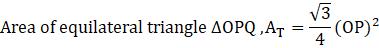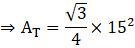AT = 97.32 sq.cm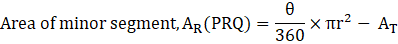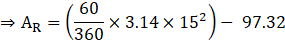⇒ AR = 117.75 – 97.32

⇒ AR = 20.43 sq.cm

Now,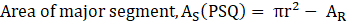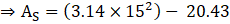⇒ AS = 706.5 – 20.43

⇒ AS = 686.07 sq.cm

∴ The area of minor segment and major segment is 20.43 sq.cm and 686.07 sq.cm respectively